# RBSE Maths Class 10 Chapter 15: Circumference of a Circle and Area Important Questions and Solutions

RBSE Class 10 Maths Chapter 15 – Circumference of a Circle and Area Important questions and solutions are provided here. All these questions are given to help the students in gaining confidence in the exam. The RBSE Class 10 important questions and solutions available at BYJU’S has a full coverage of all the concepts of Class 10.

Chapter 15 of RBSE Class 10 contains three exercises about the circumference, area, length of a sector, area of the sector of circle and combination of figures with shaded portions. These concepts are covered here with all types of possibilities of questions and solutions. Hence, these questions will help the students in scoring full marks for the questions of this chapter.

### RBSE Maths Chapter 15: Exercise 15.1 Textbook Important Questions and Solutions

Question 1: Radius of a circle is 3.5 cm. Find its circumference and area.

Solution:

Given,

Radius of the circle = r = 3.5 cm

Circumference of the circle = 2πr

= 2 × (22/7) × 3.5

= 22 cm

Area of the circle = πr2

= (22/7) × (3.5) × (3.5)

= 38.5 cm2

Therefore, circumference of the circle is 22 cm and area is 38.5 cm2.

Question 2: The circumference of a circle is 44 m. Find the area of the circle.

Solution:

Let r be the radius of the circle.

Given,

Circumference = 44 m

2πr = 44

⇒ 2 × (22/7) × r = 44

⇒ r = (44 × 7)/(2 × 22) = 7 m

Area of circle = πr2

= (22/7) × 7 × 7

= 154 m2

Hence, the area of the circle is 154 m2.

Question 3: The radius of a semi-circle shaped plot is 21 m. Find its area and perimeter.

Solution:

Given,

Radius of the semi-circular plot = r = 21 m

Area = (1/2) πr2

= (1/2) × (22/7) × 21 × 21

= 693 m2

Perimeter = (1/2) × 2πr + 2r

= πr + 2r

= (22/7) × 21 + 2 × 21

= 66 + 42

= 108 m

Therefore, the area of the plot is 693 m2 and the perimeter is 108 m.

Question 4: A wheel covers a distance of 88 metres in 100 rotations. Find the radius of the wheel.

Solution:

Given,

Wheel covered a distance of 88 m in 100 rotations

Distance covered by the wheel in 1 rotation = Circumference of the wheel

= Distance covered/Number of rotations

= 88/100 m

Let r be the radius of the wheel.

2πr = 88/100

2 × (22/7) × r = 88/100

r = (88 × 7)/(100 × 2 × 22)

= 14/100 m

= (14/100) × 100 cm

= 14 cm

Hence, the radius of the wheel is 14 cm.

Question 5: The area of a circular plate is 154 cm2. Find its circumference.

Solution:

Given,

Area of the circular plate = 154 cm2

Let r be the radius of the plate.

πr2 = 154

(22/7) × r2 = 154

r2 = (154 × 7)/22

r2 = 7 × 7

r = √(7 × 7)

r = 7 cm

Circumference of circular plate = 2πr

= 2 × (22/7) × 7

= 44 cm

Hence, the circumference of the circular plate is 44 cm.

Question 6: The circumference of a circle is equal to the perimeter of a square. If the area of the square is 484 m2, then find the area of the circle.

Solution:

Let x be the side of the square.

Thus, perimeter of square = 4x

Area of square = 484 m2 (given)

x2 = 484

x = √484 = 22 m

Perimeter of square = 4x = 4 × 22 = 88 m

Let r be the radius of circle.

According to the given,

Circumference of circle = Perimeter of square

2πr = 88

2 × (22/7) × r = 88

r = (88 × 7)/(2 × 22) = 14 m

Area of the circle = πr2

= (22/7) × 14 × 14

= 616 m2

Hence, the area of the circle is 616 m2.

Question 7: The cost of constructing a boundary wall of a circular field is Rs. 5280 at the rate of Rs. 24 per metre. Find the cost of ploughing this field at the rate of Rs. 0.50 per square metre.

Solution:

Given,

Cost of construction of boundary at the rate of Rs. 24 per metre = Rs. 5280

Let r be the radius of the circular field.

Circumference of the field = Total cost/Rate

= 5280/24

= 220

Thus, 2πr = 220

2 × (22/7) × r = 220

r = (220 × 7)/(22 × 2)

r = 35 m

Area of the field = πr2

= (22/7) × 35 × 35

= 3850 m2

Cost of ploughing the field for 1 m2 = Rs. 0.50

Total cost of ploughing = 3850 × Rs. 0.50

= Rs. 1925

Question 8: The radius of a circular grass land is 35 m. There is a footpath of width 7 m around in it. Find the area of the footpath.

Solution:

Given,

Radius of the circular grass field = 35 m.

Width of footpath = 7 m.

Radius of the field including path = R = 35 + 7 = 42 m

Area of footpath = πR2 – πr2

= π(R2 – r2)

= (22/7)[(42)2 – (35)2]

= (22/7) [(42 + 35)(42 – 35)]

= (22/7) × 77 × 7

= 1694

Hence, the area of the footpath is 1694 m2.

### RBSE Maths Chapter 15: Exercise 15.2 Textbook Important Questions and Solutions

Question 9: Radius of a circle is 7 cm and the angle subtended at the centre by an arc is 60°. Find the length of the arc.

Solution:

Given,

Radius of the circle = r = 7 cm

Angle at the centre = θ = 60°

Length of the arc = πrθ/180°

= [(22/7) × 7 × 60°]/180°

= 22/3

= 7.33

Hence, the length of the arc is 7.33 cm.

Question 10: Radius of a circle is 10.5 cm and the angle of the sector is 45°. Find the area of the minor sector of the circle. (π = 22/7)

Solution:

Given,

Radius of the circle = r = 10.5 cm

Angle at the centre = θ = 45°

Area of the minor sector = πr2θ/360°

= [(22/7) × (10.5)2 × 45°]/360°

= (22 × 10.5 × 10.5 × 45°)/(7 × 360°)

= 43.31 cm2

Therefore, the area of the minor sector is 43.31 cm2.

Question 11: The length of an arc is 12 cm and radius of a circle is 7 cm, respectively. Find the area of the minor sector of the circle.

Solution:

Given,

Length of arc of circle = l = 12 cm

Radius of the circle = r = 7 cm

Area of minor sector = (1/2)lr

= (1/2) × 12 × 7

= 42 cm2

Hence, the area of the minor sector is 42 cm2.

Question 12: The length of the minute hand of a clock is 10.5 cm. Find the area of the sector swept by the minute hand in 10 minutes. (π = 22/7)

Solution:

Given,

Length of the minute hand = r = 10.5 cm

Minute hand subtends 360° in 60 minutes (in one hour).

Angle subtended by minute hand in 10 minutes = 360°/6 = 60°

Thus, θ = 60°

Area of the sector = πr2θ/360°

= [(22/7) × (10.5)2 × 60°]/360°

= (22 × 10.5 × 10.5 × 60°)/(7 × 360°)

= 57.75 m2

Therefore, the area swept by the minute hand is 57.75 m2.

Question 13: Radius of a circle is 3.5 cm and the angle subtended by a chord at the centre is 90°. Find the area of the minor segment of the circle formed by this chord. (π = 22/7)

Solution:

Given,

Radius of circle = r = 3.5 cm

Angle subtended by the chord at the centre = 90°

Area of corresponding minor segment = (πr2θ/360°) – (1/2)r2 sin θ

= [(22/7) × 3.5 × 3.5 × 90°]/360° – (1/2) × 3.5 × 3.5 × sin 90°

= (22 × 0.5 × 3.5)/4 – (1/2) × 12.25 × 1

= (38.5)/4 – 6.125

= 9.625 – 6.125

= 3.5

Hence, the area of the minor segment is 3.5 cm2.

Question 14: Hour hand of a clock is 5 cm long. Find the area of the sector formed by this hand in 7 minutes.

Solution:

Given,

Length of the Hour hand = r = 5 cm

Hour hand subtends 360° in 12 hours.

Angle subtended by hour hand in 1 hour = 360°/12 = 30°

Angle subtended by hour hand in 1 minute = 30°/60 = (½)°

Angle subtended by hour hand in 7 minutes = (7/2)°

Thus, θ = (7/2)°

Area of the sector = πr2θ/360°

= [(22/7) × (5)2 × (7/2)°]/360°

= (22 × 25 × 42°)/(7 × 2 × 360°)

= 55/72

= 0.764 cm2

Therefore, the area required for the required sector is 0.764 cm2.

Question 15: In the figure, ABCD is a rectangle, in which side AB = 10 cm and BC = 7 cm. From the each vertex of the rectangle, four circles with radii 3.5 cm each are drawn. Find the area of the shaded portion. (π = 22/7)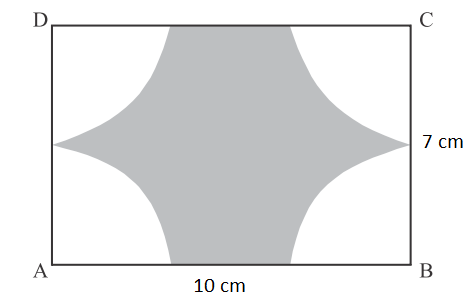Solution:

Given,

Length of the rectangle = AB = 10 cm

Width of the rectangle = BC = 7 cm

Circles are drawn at the vertices of the rectangle with radius 3.5 cm.

Angle of rectangle at the vertex = 90°

Area of sector of one vertex = πr2θ/360°

= (22/7) × 3.5 × 3.5 × 90 × (1/360°)

= 38.5/4 cm2

Area of four sectors = 4 × (38.5/4) = 38.5 cm2

Area of the shaded portion = Area of rectangle – Area of four sectors

= 10 × 7 – 38.5

= 70 – 38.5

= 31.5 cm2

Therefore, the area of the shaded portion is 31.5 cm2.

### RBSE Maths Chapter 15: Exercise 15.3 Textbook Important Questions and Solutions

Question 16: Find the circumference of the incircle of square with side 14 cm.

Solution:

Given,

Side of a square = 14 cm

Diameter of circle = Side of the square = 14 cm

Radius of the circle = r = 14/2 = 7 cm

Circumference of the circle = 2πr

= 2 × (22/7) × 7

= 44 cm

Hence, the circumference of the circle inscribed in a square is 44 cm.

Question 17: The difference between the circumference and the radius of a circle is 74 cm, find the area of the circle.

Solution:

Let r be the radius of the circle.

According to the given,

Circumference of the circle – Radius = 74 cm

2πr – r = 74

r(2π – 1) = 74

r[2 × (22/7) – 1] = 74

r[(44 – 7)/7] = 74

r(37/7) = 74

r = (74 × 7)/37

r = 14 cm

Area of the circle = πr2

= (22/7) × 14 × 14

= 616 cm2

Question 18: In the figure, O is the centre of a circle. ∠AOB = 90° and OA = 3 cm, then find the area of the shaded region.Solution:

Given,

Radius of circle = r = 3 cm

Angle subtended by the chord at the centre = 90°

Area of the shaded region = (πr2θ/360°) – (1/2)r2 sin θ

= [(22/7) × 3 × 3 × 90°]/360° – (1/2) × 3 × 3 × sin 90°

= [(22 × 9)/ (7 × 4)] – (1/2) × 9 × 1

= (99/14) – (9/2)

= 7.07 – 4.5

= 2.57 cm2

Hence, the area of the shaded region is 2.57 cm2.

Question 19: If the perimeter of a circle is equal to the perimeter of a square, then find the ratio of their areas.

Solution:

Let r be the radius of the circle.

And a be the side of the square.

According to the given,

Perimeter of circle = Perimeter of square

2πr = 4a

r/a = 4/2π

r/a = 2/π ….(i)

Area of circle/Area of square = πr2/a2

= π(r/a)2

= π(2/π)2 [From (i)]

= 4/π

= (4 × 7)/22

= 28/22

= 14/11

Hence, the required ratio is 14 : 11.

Question 20: The radius of a circular park is 3.5 m. There is a footpath (see in the figure) of width 1.4 m around the park. Find the area of the footpath.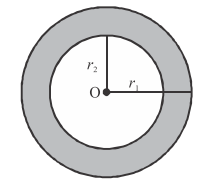Solution:

From the given,

r = 3.5 cm

Width of the footpath = 1.4 m

R = 3.5 + 1.4 = 4.9 m

Area of the footpath = π(R2 – r2)

= (22/7) [(4.9)2 – (3.5)2]

= (22/7) [(4.9 + 3.5)(4.9 – 3.5)]

= (22/7) × 8.4 × 1.4

= 22 × 1.2 × 1.4

= 36.96 m2

Therefore, the area of the footpath is 36.96 m2.

Question 21: Find the area of the square inscribed in a circle of radius 8 cm.

Solution:

Let a be the side of the square.

Given,

Radius of the circle = r = 8 cm

Diameter of circle = 2r = 2(8) = 16 cm

Given that, the square is inscribed in a circle.

Diameter of circle = Diagonal of square

16 = a√2

a = 16/√2

a = (16/√2) × (√2/√2)

a = (16√2)/2

a = 8√2 cm

Area of square = a2

= (8√2)2

= 64 × (2)

= 128 cm2

Hence, the area of the square is 128 cm2.

Question 22: In the given figure, AB is the diameter of a circle, AC = 6 cm and BC = 8 cm. Find the area of the shaded portion.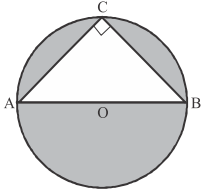Solution:

Given,

O is the centre and AB is the diameter of the circle.

Angle in a semicircle = 90°

In right triangle ACB,

By Pythagoras theorem,

AB2 = AC2 + BC2

AB2 = (6)2 + (8)2

= 36 + 64

= 100

AB = √100 = 10 cm

Therefore, diameter of the circle = 10 cm

Thus, radius of the circle = r = 10/2 = 5 cm

Area of the circle = πr2

= (22/7) × 5 × 5

= 550/7

= 78.57 cm2

Area of right triangle ACB = (1/2) × base × height

= (1/2) × 6 × 8

= 24 cm2

Area of the shaded region = Area of circle – Area of triangle ACB

= 78.57 – 24

= 54.57 cm2

Question 23: Find the area of the shaded design of the given figure, where ABCD is a square with a side 10 cm and taking every side of the square as the diameter, four semicircles are drawn. (π = 3.14)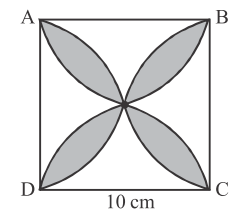Solution:

Given,

Side of the square = 10 cm

Let us mark the unshaded parts as I, II, III and IV and mark O where these parts meet each other.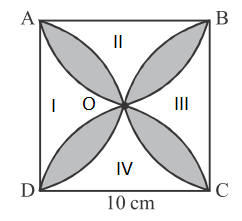Area of part I and III = Area of square ABCD – [area of semicircle AOB + area of semicircle COD]

= (10 × 10) – [(1/2) × 3.14 × 5 × 5 + (1/2) × 3.14 × 5 × 5]

= 100 – (3.14 × 25)

= 100 – 78.5

= 21.5 cm2

Similarly,

Area of part II and IV = 21.5 cm2

Area of the shaded design = Area of square ABCD – Area of part (I + II + III + IV)

= 100 – (21.5 + 21.5)

= 100 – 43

= 57 cm2

Question 24: In the given figure, the radius of the semicircle is 7 cm. Find the area of the circle formed inside the semicircle.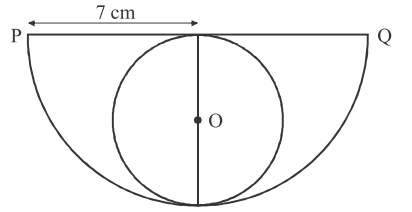Solution:

Given,

Radius of semicircle = 7 cm

From the given figure,

Diameter of circle formed inside the semicircle = Radius of semicircle = 7 cm

Radius of circle formed insider the semicircle = r = 7/2 = 3.5 cm

Area of the circle formed inside of a semicircle = πr2

= (22/7) × 3.5 × 3.5

= 38.5

Hence, the area of required circle is 38.5 cm2

### RBSE Maths Chapter 15: Additional Important Questions and Solutions

Question 1: The area between two concentric circles will be

(A) πR2

(B) π(R + r)(R – r)

(C) π(R2 – r)

(D) None of these

Solution:

Let R and r be the radii of two concentric circles as shown below.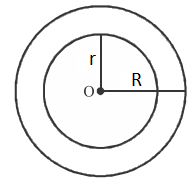Area of outer circle = πR2

Area of inner circle = πr2

Area between concentric circles = πR2 – πr2

= π(R2 – r2)

= π(R + r)(R – r)

Question 2: The radii of two concentric circles are 4 cm and 3 cm. The area between these two circles will be:

(A) 22 cm2

(B) 12 cm2

(C) 32 cm2

(D) 18 cm2

Solution:

Let the radii of two concentric circles be R = 4 cm and r = 3 cm.

We know that area between two concentric circles = π(R2 – r2)

= (22/7) [(4)2 – (3)2]

= (22/7) × (16 – 9)

= (22/7) × 7

= 22 cm2

Question 3: The sum of the circumferences of two circles with radii R1 and R2 is equal to the perimeter of the circle with radius R. Choose the correct option.

(A) R1 + R2 = R

(B) R1 + R2 > R

(C) R1 + R2 < R

(D) Nothing can be said

Solution:

Given,

The sum of circumferences of two circles with radii R1 and R2 is equal to the perimeter of the circle with radius R.

Thus, 2πR1 +2 πR2 = 2πR

2π(R1 + R2) = 2πR

⇒ R1 + R2 = R

Question 4: Radius of a circle is 9 cm and the angle of the sector is 70°. Find the area of the minor sector of the circle. (π = 22/7)

Solution:

Given,

Radius of the circle = r = 9 cm

Angle at the centre = θ = 70°

Area of the minor sector = πr2θ/360°

= [(22/7) × (9)2 × 70°]/360°

= (22 × 9 × 9 × 10°)/360°

= 99/2

= 49.5 cm2

Therefore, the area of the minor sector is 49.5 cm2.

Question 5: If an arc of a circle subtends an angle of 60° at the centre and if the area of the minor sector is 231 cm2, then find the radius of the circle.

Solution:

Let r be the radius of the circle.

Given,

Angle at the centre = θ = 60°

Area of the minor sector = 231 cm2

πr2θ/360° = 231

[(22/7) × r2 × 60°]/360° = 231

(22 × r2)/(7 × 6) = 231

r2 = (231 × 7 × 6)/22

r2 = 21 × 7 × 3

r2 = 21 × 21

r = √(21 × 21)

r = 21 cm

Hence, the radius of the circle is 21 cm.

Question 6: Find the radius of the circle whose area is 616 cm2.

Solution:

Let r be the radius of the circle.

Given,

Area of the circle = 616 cm2

πr2 = 616

(22/7) × r2 = 616

r2 = (616 × 7)/22

r2 = 28 × 7

r2 = 196

r2 = √196 = 14

Therefore, the radius of the circle is 14 cm.

Question 7: Write the length of an arc of a sector of circle with radius r and angle with degree measure θ.

Solution:

If r be the radius and θ be the angle at the centre of the circle made by the sector, then the length of an arc of a sector is given by:

L = 2πrθ/360°

Or

L = πrθ/180°

Question 8: Find the circumference of a circle whose diameter is 14 cm.

Solution:

Given,

Diameter of the circle = d = 14 cm

Radius of the circle = r = d/2

= 14/2 = 7 cm

Circumference of the circle = 2πr

= 2 × (22/7) × 7

= 22 × 2

= 44 cm

Hence, the circumference of the given circle is 44 cm.

Question 9: Find the area of the corresponding major sector of a circle with radius 7 cm and angle 120°.

Solution:

Given,

Radius of the circle = r = 7 cm

Angle at the centre = θ = 120°

Area of the minor sector = πr2θ/360°

= [(22/7) × (7)2 × 120°]/360°

= (22 × 7 × 7)/(7 × 3)

= 154/3 cm2

Area of the circle = πr2

= (22/7) × 7 × 7

= 154 cm2

Area of the corresponding major sector = Area of circle – Area of the minor sector

= 154 – (154/3)

= 154[1 – (1/3)]

= 154 × (2/3)

= 308/3

= 102.67 cm2 (approx)